# HC Verma Solutions Class 11 Chapter 13 Fluid Mechanics

HC Verma Class 11 Solutions Chapter 13 Fluid Mechanics deals with problems based on finding the pressure of the fluids and more. The chapter consists of questions that are based on finding the pressure of water if the tap is open or closed and finding the pressure at the bottom of a tube when it is attached to a gas chamber etc. Students will further get to learn topics of fluid mechanics and solve problems like:

• Finding the height if pressure, area, mass are given.
• Problems related to standard atmospheric pressure and finding the torque and force exerted by flowing water on rocks.
• There are questions where students need to find the amount of copper in an ornament when the volume of the ornament is given as well as calculate the volume of a boat filled with water inside using the concept of buoyant force.

In order to help students understand the concept clearly and be proficient in solving the question from the given chapter, we are offering HC Verma solutions for part 1 that will help them master physics concepts and be well prepared for JEE Main and Advanced.

In chapter 13 students will learn about important topics like;

1. Fluids
2. Pressure in a Fluid
3. Pascal’s Law
4. Atmospheric Pressure and Barometer
5. Archimedes’ Principle
6. Pressure Difference and Buoyant
7. Force in Accelerating Fluids
8. Flow of Fluids
10. Irrotational Flow of an
11. Incompressible and Nonviscous Fluid
12. Equation of Continuity
13. Bernoulli’s Equation
14. Applications of Bernoulli’s Equation

## Class 11 Important Questions In Chapter 13

1. A barometer tube has a reading of 76 cm of mercury. The tube is slowly moved to an inclined position while the open end is kept immersed in the mercury reservoir. State whether the length of the mercury column will be 76 cm or less than 76 cm or more than 76 cm?

2. A small amount of water slowly gushes out of a vertical pipe. However, as it descends its area of cross-section reduces. Can you explain this event on the basis of the equation of continuity?

3. State what is Bernoulli’s theorem based on;

(a) conservation of momentum (b) conservation of mass (c) conservation of energy (d) conservation of angular momentum

4. We take an empty vessel and place a metal cube in it. Water is then filled in the vessel and the cube is completely immersed in the water. What will happen to the force on the bottom of the vessel in contact with the cube?

(a) It will increase (b) Decreases (c) Remains the same (d) Becomes zero.

5. An open tank filled with a liquid has a small hole near the bottom. As the water is released, what will the speed of the water not depend upon?

(a) The density of the liquid (b) Height of the liquid from the hole (c) Area of the hole (d) Acceleration due to gravity

## HC Verma Solutions Vol 1 Fluid Mechanics Chapter 13

Question 1: The surface of water in a water tank on the top of a house is 4 m above the tap level. Find the pressure of water at the tap when the tap is closed, is it necessary to specify that the tap is closed? Take g = 10 m s-2.

Solution:

Pressure = P = ρgh

Here ρ = 1000 kg/m3, g = 10 m s-2 and h = 4 m

=> P = 40000 N/m2

Yes, it necessary to state that the tap is closed. If tap is open the level of water will decrease and hence the pressure will decrease, the pressure at the tap is atmospheric.

Question 2: The heights of mercury surfaces in the two arms of the manometer shown in figure (below) are 2 cm and 8 cm. Atmospheric pressure = 101 × 10 N m-2. Find (a) the pressure of the gas in the cylinder and (b) the pressure of mercury at the bottom of the U tube.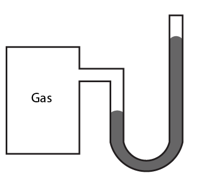Solution: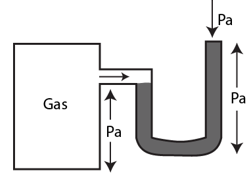(a) Pressure at the bottom of the tubes should be same when considered for both limbs.

From figure,

Pa + ρHg h1 g = Pg + ρHg h2 g

=> Pg = Pa + ρHg g(h1 – h2)

(b) Pressure of mercury at the bottom of tube

P = Pa + ρHg h1 g

Question 3: The area of cross section of the wider tube shown in figure (below) is 900 cm2. If the boy standing on the piston weighs 45 kg, find the difference in the levels of water in the two tubes.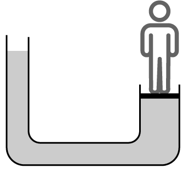Solution:

Pressure = Force/Area

F = mg = 45 x 9.8 = 441 N

and A = 900 cm2 or 0.09 m2

Therefore, Pressure = 441/ 0.09 = 4900 N …(1)

Also, pressure can be represented as, P = ρg Δh

Where, Δh is the height difference and ρ = density

Density of water = ρ = 1000 kg/m3

=> P = 1000 x 9.8 x Δh …(2)

from (1) and (2)

4900 = 1000 x 9.8 x Δh

or Δh = 50 cm

Question 4: A glass full of water has a bottom of area 20 cm2, top of area 20 cm2, height 20 cm and volume half a liter. (a) Find the force exerted by the water on the bottom. (b) Considering the equilibrium of the water, find the resultant force exerted by the sides of the glass on the water. Atmospheric pressure = 10 × 105 N m-2. Density of water = 1000 kg m-3 and g = 10 m s-2. Take all numbers to be exact.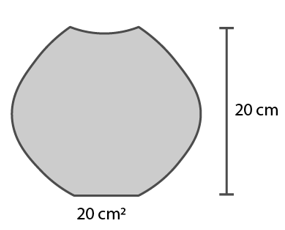Solution:

(a) Pressure at bottom of the container = pressure due to water + atmospheric pressure.

P = ρg h + Patm

= (1000 x 10 x 0.2) + 1 x 105

= 1.02 x 105 N/m2

Now, Force exerted on the bottom of the container = F = Px Area

= (1.02 x 105) x 0.002

F = 204 N

(b) Vertical forces (F’) includes the weight of the water and the force due to atmospheric pressure.

F’ = mg + [P_atm x area]

= (0.5 x 9.8) + (0.002 x 1 x 10^5)

F’ = 205 N

Therefore, total upward force = F’ – F = 1 N

Question 5: Suppose the glass of the previous problem is covered by a jar and the air inside the jar is completely pumped out. (a) What will be the answers to the problem? (b) Show that the answers do not change if a glass of different shape is used provided the height, the bottom area and the volume are unchanged.

Solution:

If the glass is covered by a jar and the air inside the jar is completely pumped out.

Pressure at the bottom of the glass = P = ρ gh = 1000 x 10 x 0.2 = 2000 N/m2

The downward force is F = P x area = 2000 x 0.002 = 4N

(b) Vertical force is equal to the weight of the water = mg = 0.5 x 10 = 5N

The horizontal forces again cancel out. So the total upward force = 1N

It glass of different shape is used provided the volume, height and area remain same, no change in answer will occur.

Question 6: If water be used to construct a barometer, what would be the height of water column at standard atmospheric pressure (76 cm of mercury)?

Solution:

Standard atmospheric pressure is always pressure exerted by 76 cm Hg column.

Patm|Hg = ρHg gh = 13.6 x 103 x g x 0.76 …(1)

For water, Patm|water = ρwater gh = 1000 x g x 0.76 …(2)

Atmospheric pressure: P_atm|Hg = P_atm|water

equating both the equations, we get

h = 1033.6 cm, is the required height of the water.

Question 7: Find the force exerted by the water on a 2 m2 plane surface of a large stone placed at the bottom of a sea 500 m deep. Does the force depend on the orientation of the surface?

Solution:

Pressure = P = ρgh = 1000 x 10 x 500 = 5 x 106 N/m2

and force = F = Pressure x Area = (5 x 106) x 2 = 107 N

Question 8: Water is filled in a rectangular tank of size 3 m × 2 m × 1 m. (a) Find the total force exerted by the water on the bottom surface of the tank. (b) Consider a vertical side of area 2 m × 1 m. Take a horizontal strip of width δx meter in this side, situated at a depth of x meter from the surface of water. Find the force by the water on this strip (c) Find the torque of the force calculated in part (b) about the bottom edge of this side. (d) Find the total force by the water on this side. (e) Find the total torque by the water on the side about the bottom edge. Neglect the atmospheric pressure and take g = 10 m s-2.

Solution:

Dimensions of rectangular tank : 3 m × 2 m × 1 m.

(a) Pressure at the bottom of the tank = P = ρgh

= 1000 x 10 x 1 = 104 N/m2

Area of the bottom of the tank = A = lb = 3×2 = 6m2

Now, force = F = Pressure x Area = 104 x 6 = 60000 N

(b) P = ρgh = ρgx

Area of strip = A = 2 dx

So, the force on the strip = F = PA = ρgx x 2 dx

= (20000xdx) N

(c) Let us first find the perpendicular distance(d) of the strip from the bottom edge.

d = h-x = (1-x) m

Now, Torque = Force x perpendicular distance = Fd

= (20000x(1-x)dx) N-m

(d) Total force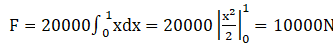(e) Total Torque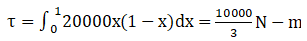Question 9: An ornament weighing 36 g in air, weighs only 34 g in water. Assuming that some copper is mixed with gold to prepare the ornament, find the amount of copper in it. Specific gravity of gold is 19.3 and that of copper is 8.9.

Solution: The density of copper and gold are 8.9 and 19.3 respectively.

V = x/8.9 + (36-x)/19.3 …(1)

When ornament placed in water, it displaces water equal to its weight. The density of water = ρwater = 1 g/cm3

Weight of the water displaced = w = mg = v ρwater g = vg ….(2)

ornament weighs 34 g in water.(Given)

The buoyant force = Fb = mg = (36-34) = 2g …(3)

Buoyant force is equal to weight of the water displaced.

vg = 2g

=> [x/8.9 + (36-x)/19.3] g = 2g

or x = 23.14/10.4 = 2.22 grams (approx)

The weight of copper in the ornament is 2.22 grams.

Question 10: Refer to the previous problem. Suppose, the goldsmith argues that he has not mixed copper or any other material with gold, rather some cavities might have been left inside the ornament. Calculate the volume of the cavities left that will allow the weights given in that problem.

Solution:

Total volume of the Ornament(y)= Volume of gold + volume of cavity

Total volume (y)= 36/19.3 +v

Using, Principle of Flotation,

The volume of the water displaced is also y cm3

The mass of the water displaced =y grams

The weight of the water displaced = yg

yg = 2g

or y = 2

Therefore, 36/19.3 +v = 2

or v = 0.13 cm3

Question 11: A metal piece of mass 160 g lies in equilibrium inside a glass of water (figure below). The piece touches the bottom of the glass at a small number of points. If the density of the metal is 8000 kg m4, find the normal force exerted by the bottom of the glass on the metal piece.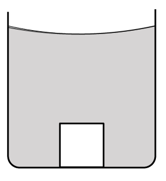Solution:

Volume of metal immersed, = V = m/ρ = 0.16/8000 = 0.00002 m3

Weight of the water displaced = Fb = V x ρwater x g

= 0.00002 x 1000 x 10

= 0.2

Find the normal force exerted by the bottom of the glass on the metal piece:

Vertical forces consist of the normal reaction(N) from the surface (upwards), the weight of the metal(downwards) and the buoyant force(upwards) to maintain the balance of vertical forces.

N + F_b = mg

=> N = (0.16×10)-0.2 = 1.4 N

The normal reaction is 1/4 N in total.

Question 12: A ferry boat has internal volume 1 m3 and weight 50 kg. (a) Neglecting the thickness of the wood, find the fraction of the volume of the boat immersed in water. (b) If a leak develops in the bottom and water starts coming in, what fraction of the boat’s volume will be filled with water before water starts coming in from the sides?

Solution:

(a) Buoyant force should be equal to the weight of the boat.

vw g = mg

v x 1000 = 50

or v = 1/20 m3

(b) Let V_b be the volume of boat filled with water before water starts coming in form the sides. here Vb 1 m3

Therefore, buoyant force = Fb = Vb ρw g = 1000 gN

The volume of water = Vw = mww = 950/1000 = 0.95 m3

[Here mw + 50 = 1000 or mw = 950 kg]

Now, fraction of water filled in the boat = 0.95 = 19/20

Question 13: A cubical block of ice floating in water has to support a metal piece weighing 0.5 kg. What can be the minimum edge of the block so that it does not sink in water? Specific gravity of ice 0.9.

Solution:

Let the edge of the cube be “x cm”.

Volume of the cube = V = x3/106 m3 …(1)

And buoyant force = Fb = mmg + V ρi g = 0.5 + 900 v …(2)

Also, buoyant force is equal to the weight of the water displaced

=> V x 1000 g = 0.5 + 900

=> 100V = 0.5

Using (1),

x3/106 = 0.5/100

or x = 17 cm (approx)

Question 14: A cube of ice floats partly in water and partly in K.oil (figure below). Find the ratio of the volume of ice immersed in water to that in K.oil. Specific gravity of K.oil is 0.8 and that of ice is 0.9.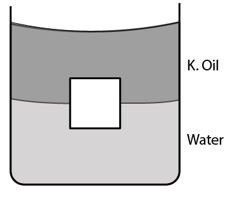Solution:

Density of water = ρw = 1000 kg/m3

Density of ice = ρi = 0.9 x 1000 = 900 kg/m3

Density of K.oil = ρo = 1000 kg/m3

The weight of the cube of ice = wi = volume x density x g = (a+b)900g …(1)

Where a = volume of cube immersed in water and b = volume immersed in K.oil

The total buoyant force = sum of forces exerted by the liquids.

=> Fb = a ρw + bρo= a(1000) + b(800) ….(2)

From (1) and (2)

a(1000) + b(800) = (a+b)900

=> a/b = 1

The ratio of the volumes immersed is 1:1.

Question 15: A cubical box is to be constructed with iron sheets 1 mm in thickness. What can be the minimum value of the external edge so that the cube does not sink in Water? Density of iron = 8000 kg m-3 and density of water = 1000 kg m-3.

Solution:

Density of iron = ρi = 8000 kg/m3 and density of water = 1000 kg m-3.

Let “x cm” be the edge of the iron sheet.

Surface area = 6x2 cm2

Volume of the iron sheets = v = 6x2 x 0.1 = (0.6/106) m3

Therefore, mass of the iron box= mb = ρi x v = 4.8 x 10-3 x2 kg …(1)

volume of the cube = V’ = x3 cm3 = (x3/106) m3

buoyant force = Fb = V’ ρw g

= (x3/106) x 1000 x g

= (x3/103) gN …..(2)

Here, buoyant force is equal to the weight of the box.

From (1) and (2)

4.8 x 10-3 x2 = (x3/103)g

=> x = 4.8 cm

Question 16: A cubical block of wood weighing 200 g has a lead piece fastened underneath. Find the mass of the lead piece which will just allow the block to float in water. Specific gravity of wood is 0’S and that of lead is 11.3.

Solution :

Total mass of the system = mass of wood (mw) + mass of lead(mPb)

= 200 + mPb …(1)

Density of wood = ρw = 0.8 g/cm3

Volume of the wooden block = vw = mww = 250 cm3

Density of lead = ρPb = 11.3 g/cm3

Volume of lead piece = vPb = mPbPb = (mPb/11.3) cm3

Therefore, the volume of water displaced would be equal to the total volume.

V = vw + vPb = 250 + (mPb/11.3) cm3

Fb = v ρw g = (250 + mPb/11.3) x 1 x g ….(2)

Now, (1) = (2), we get

200 + mPb = (250 + mPb/11.3) x 1 x g

=> mPb = 54.8 g

Question 17: Solve the previous problem if the lead piece is fastened on the top surface of the block and the block is to float with its upper surface just dipping into water.

Solution:

volume immersed is only due to the wooden block.

The buoyant force = Fb = 250g

Now,

Total mass = Fb

=> 200 + mPb = 250 g

or mPb = 50 g

The mass of the lead piece is 50 g.

Question 18: A cubical metal block of edge 12 cm floats in mercury with one fifth of the height inside the mercury. Water is poured till the surface of the block is just immersed in it. Find the height of the water column to be poured. Specific gravity of mercury = 13.6.

Solution:

Volume immersed in mercury= vm = (123/5) cm3

Buoyant force on the block is equal to the weight of the mercury displaced = ρm x vm

= 13.6 x (123/5) g ….(1)

Volume of block in water = vw = 123 h cm3

Volume of block in mercury = v’m = 122(12-h) cm3

Now, buoyant force = Fb = [vm ρw + vm‘ ρm]g

= 122 h + 122 (12-h)13.6 g …..(2)

The buoyant force is equal to the weight of the cube.

(1)=(2)

=> 13.6 x (123/5) g = 122 h + 122 (12-h)13.6 g

Solving above equation, we have

h = 10.4 cm

Therefore, water needs to be poured to a height of 10.4 cm.

Question 19: A hollow spherical body of inner and outer radii 6 cm and 8 cm respectively floats half-submerged in water. Find the density of the material of the sphere.

Solution:

A hollow spherical body of inner and outer radii 6 cm and 8 cm respectively floats half-submerged in water.

Find density of the material of the sphere:

Here, Mass of the water displaced = Mass of the material,

ρw x Vi = vb x ρ

=> 1000 x (2/3) π 83 = (4/3)π (83 – 63

Solving above equation, we have

ρ = 865 kg/m3

Question 20: A solid sphere of radius 5 cm floats in water. If a maximum load of 0.1 kg can be put on it without wetting the load, find the specific gravity of the material of the sphere.

Solution:

A solid sphere of radius 5 cm floats in water. If a maximum load of 0.1 kg can be put on it without wetting the load

Volume of sphere = v = 4πr3/3 = (5π/3) x 10-4 m3

Weight of water displaced = ρw vg = (5π/3) N

Now, Weight of sphere + Load = weight of displaced water

ρs vg + 0.1 x 10 = ρw vg

or ρs = [ρw vg – 1]/vg

Therefore, specific gravity = ρsw = 1 – 1/[5π/3] = 0.81

Which is the specific gravity of sphere.

Question 21: Find the ratio of the weights, as measured by a spring balance, of a 1 kg block of iron and a 1 kg block of wood. Density of iron = 7800 kg m-3, density of wood = 800 kg m-3 and density of air = 1293 kg m-3.

Solution:

The weight of iron block = wi = mi g – vi ρa g = mi [1 – (1/ρi) ρa]g

and weight of wooden block = ww = mw g – vw ρa g = mw [1 – (1/ρw) ρa]g

Now, ratio of wi and ww: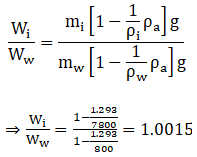Question 22: A cylindrical object of outer diameter 20 cm and mass 2 kg floats in water with its axis vertical. If it is slightly depressed and then released, find the time period of the resulting simple harmonic motion of the object.

Solution:

Buoyant force = Fb = v ρw g = (π r2 x)ρw g

Where v = volume of the immersed object

and ma = (π r2 x)ρw g

or a = (π r2 x ρw g)/2000

Now, time period = T = 2π √(displacement/acceleration)

=> T = 0.5 sec (approx)

Question 23: A cylindrical object of outer diameter 10 cm, height 20 cm and density 8000 kg m-3 is supported by a vertical spring and is half dipped in water as shown in figure(below). (a) Find the elongation of the spring in equilibrium condition. (b) 1f the object is slightly depressed and released, find the time period of resulting oscillations of the object. The spring constant =500 N m-1.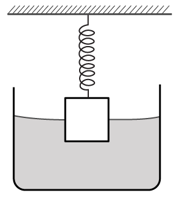Solution:

(a)In the equilibrium condition, the weight of the cylinder, is supported by the spring and the buoyant force.

kx + v ρw g = mg

where v is volume = πr2(h/2)

=> 500x + (π(0.05) 2 x0.1x10x1000) = π((0.05) 2 x 0.2x8000x10)

Solving above equation, we get

x = 23.5 cm

(b) Driving force = F = kx + vρw g

=> ma = kx + πr2 x ρw g

=> a = 2π √(displacement/acceleration)/m

Time period, T = 2π √(displacement/acceleration)

= 2π √0.5x/[500+(π(0.05) 2 x1000x10)x]

= 0.935 sec

Question 24: A wooden block of mass 0.5 kg and density 800 kg m-3 is fastened to the free end of a vertical spring of spring constant 50 N m-1 fixed at the bottom. If the entire system is completely immersed in water find (a) the elongation (for compression) of the spring in equilibrium and (b) the time-period of vertical oscillations of the block when it is slightly depressed and released.

Solution:

(a) The weight of the block is balanced by the spring and the buoyant force.

=> mg = kx + v ρw g

=> 0.5 x 10 = 50x + (0.5/800) x 1000 x 10

Solving for x,

x = 2.5 cm

(b) a = kx/m

w2x = kx/m

Time period = T = 2π √(m/k) = (π/5) sec

Question 25: A cube of ice of edge 4 cm is placed in an empty cylindrical glass of inner diameter 6 cm. Assume that the ice melts uniformly from each side so that it always retains its cubical shape. Remembering that ice is lighter than water, find the length of the edge of the ice cube at the instant it just leaves contact with the bottom of the glass.

Solution:

The weight of the remaining ice will be balanced by the buoyant force provided from the melted water.

mg = v ρw g

The mass of the ice = m = x3 x ρi

=> x3 x 0.9 = x2 x h x 1

=> h = 0.9 x

Volume of water formed = 43 – x3 = πr2 h – x2 h

x = 2.26 cm

Question 26: A U-tube containing a liquid is accelerated horizontally with a constant acceleration αo. If the separation between the vertical limbs is l, find the difference in the heights of the liquid in the two arms.

Solution:

Balance all the vertical forces on the tube.

Pa A + Alphao = Pa + Ahρg

Where Pa is atmospheric pressure.

=> ao I = hg

or h = a0/g

Question 27: At Deoprayag (Garhwal, UP) river Alaknanda mixes with the river Bhagjrathi and becomes river Ganga. Suppose Alaknanda has a width of 12 m, Bhagirathi has a width of 8 m and Ganga has a width of 16 m. Assume that the depth of water is same in the three rivers. Let the average speed of water in Alaknanda be 20 km h-1 and in Bhagirathi be 16 km h-1. Find the average speed of water in the river Ganga.

Solution:

The sum of the volume flow from Alaknanda and Bhagirathi is equal to the volume flow in Ganga.

Let the depth of the rivers be “d”

vA x 12 x d + vB x 8 x d = vG x 16 x d

=> vG = 23 km/h

Question 28: Water flows through a horizontal tube of variable cross section (figure below). The area of cross section at A and B are 4 mm2 and 2 mm2 respectively. If 1 cc of water enters per second through A, find (a) the speed of water at A, (b) the speed of water at B and (c) the pressure difference PA – PB.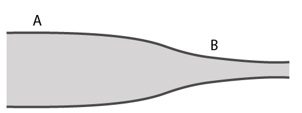Solution:

(a)The total volume is equal to area times velocity.

vA x av = v

Where vA is the velocity in tube.

=> 4 x 10-2 x vA = 1

=> VA = 25 cm/s

(b) Let the velocity in tube B, vB

For steady flow: vA x aA = vB x aB

=> 25 x 4 x 10-2 = vB x 2 x 10-2

=> VB = 50 cm/s

(c) From Bernoulli equation,

PA + (1/2)ρ vA2 = PB + (1/2) ρ VB2

=> PA – PB = (1/2)ρ [vB2 – vA2]

= (1/2) x 1000 x (0.52 – 0.252)

= 94 N/m2

Question 29: Suppose the tube in the previous problem is kept vertical with A upward but the other conditions remain the same. The Separation between the cross sections at A and B is 15/16 cm. Repeat parts (a), (b) and (c) of the previous problem. Take g = 10 m s-2.

Solution:

VA = 25 cm/s and VB = 50 cm/s [from previous problem solution]

As changing the orientation doesn’t change the volume of water flowing from the tubes.

(c) From Bernoulli’s equation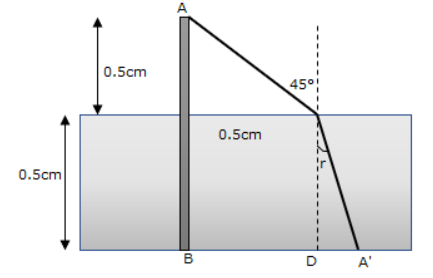Therefore, pressure difference is 0 N/m2

Question 30: Suppose the tube in the previous problem is kept vertical with B upward. Water enters through B at the rate of 1 cm3 s-1. Repeat parts (a), (b) and (c). Note that the Speed decreases as the water falls down.

Solution:

(a)The total volume is equal to area times velocity.

V = aB x vB

Where, v = 1 cm3, aA = area of cross-section of tube A = 4 mm2 and aB = area of cross-section of tube B = 2 mm2

=> vB = 50 cm/s

(b) let vb be the velocity in tube

vA x aA = vB x aB

=> vA = 25 cm/s

(c) From Bernoulli equationQuestion 31: Water flows through a tube shown in figure (below). The areas of cross section at A and B are 1 cm2 and 0.5 cm2 respectively. The height difference between A and B is 5 Cm. If the speed of water at A is 10 cm s-1, find (a) the speed at B and (b) the difference in pressures at A and B.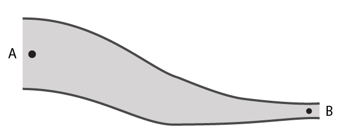Solution:

(a)From equation of continuity,

vA x aA = vB x av

0.1 x 1 = 0.5 x vB

=> vB = 20cm/s

(b) From Bernoulli’s equation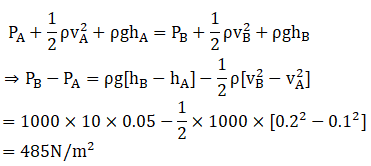Question 32: Water flows through a horizontal tube as shown in figure (below). If the difference of heights of water column in the vertical tubes is 2 cm, and the areas of cross section at A and B are 4 cm2 and 2 cm2 respectively, find the rate of flow of water across any section.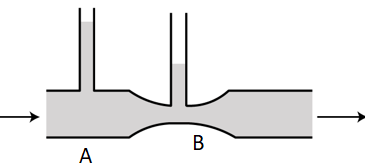Solution:

Equation of continuity: vA x aA = vB x aB

=> vB = 2 vA

Where vA and vB speed of water at A and speed of water at B respectively.

aA = Area of cross-section of tube A and aB = Area of cross-section of tube B

Also, given h = difference in height in the two columns = 2 cm

Pressure difference between A and B: PA – PB = ρ gh = 1000 x 10 x 0.02 = 200 N/m2

By Bernoulli’s equation,

PA + (1/2)ρ[vB2 – vA2]

200 = (1/2) x 1000 x [4vA2 – vA2]

or vA2 = 400/(3×100)

or vA = 36.51 cm/s

Rate of flow = vA x aA = 36.51 x 4 = 146 cm3/s (approx)

Question 33: Water flows through the tube shown in figure (below). The areas of cross section of the wide and the narrow portions of the tube are 5 cm2 and 2 cm2 respectively. The rate of flow of water through the tube is 500 cm3 s-1. Find the difference of mercury levels in the U-tube.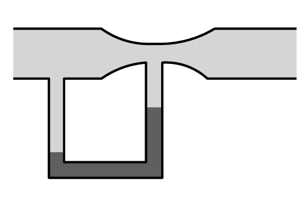Solution:

Equation of continuity: vA x aA = vB x aB

Where vA and vB are velocities of flow at A and B

vA x 5 = 500

=> vA = 100 cm/s or 1 m/s

Similarly, vB = 250 cm/s or 2.5 m/s

Now, the pressure difference between the points,

PA – PB = ρHg gh = 13.6 x 10 x h

From the Bernoulli’s equation: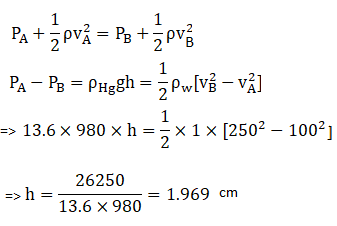Question 34: Water leaks out from an open tank through a hole of area 2 mm2 in the bottom. Suppose water is filled up to a height of 80 cm and the area of cross section of the tank is 0.4 m2. The pressure at the open surface and at the hole are equal to the atmospheric pressure. Neglect the small velocity of the water near the open surface in the tank. (a) Find the initial speed of water coming out of the hole. (b) Find the speed of water coming out when half of water has leaked out. (c) Find the volume of water leaked out during a time interval dt after the height remained is h. Thus, find the decrease in height dh in terms of h and dt. (d) From the result of part (e) find the time required for half of the water to leak out.

Solution:

(a) The velocity of water exiting at “a”

vA = √2gh

=> vA = √(2x10x9.8) = 4 m/s

(b) vB = √(2g.h/2) = √(2x10x0.4) = √8 m/s

(c) volume flow = V = Area x height = Ah

Therefore, dv/dt = A dh/dt …(1)

We know, V = av dt

or V = a (√2gh) t

Differentiating above equation, we have

dV/dt = A x √2gh …(2)

From (1) and (2)

a (√2gh) = A dh/dt

=> 2 x 10-6 (√2gh) = 0.4 dh/dt

=> dh/(√2gh) = 5×10-6 dt

(d) integrating above equation ,we have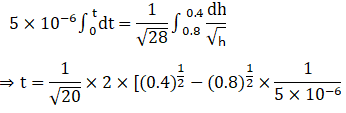solving for t,

t = 6.51 h

Question 35: Water level is maintained in a cylindrical vessel up to a fixed height H. The vessel is kept on a horizontal plane. At what height above the bottom should a hole be made in the vessel so that the water stream coming out of the hole strikes the horizontal plane at the greatest distance from the vessel (figure below)?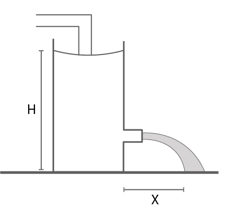Solution 35: Water level is maintained in a cylindrical vessel up to a fixed height H.

Height of water above the hole = H-h

Velocity with which water = v = √[2g[H-h] and time of flight be t

t = √(2h/g)

The horizontal distance travelled = x = vt = √[2g[H-h] x √(2h/g)

=> x = √[4[Hh-h2]

To maximize this function: dx/dh = 0

d/dh √[4[Hh-h2] = 0

h = H/2